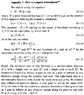# How to prove Coleman's formula for the ratio of two determinants?

• omg!
In summary, the author is trying to prove the theorem found in the following scan of Coleman's Aspects of Symmetry. So far, they have managed to show that the functions on both sides have simple zeros/poles, but they fail to see why they are meromorphic functions, and the steps that lead to their asymptotic behavior. Regarding asymptotics, they have shown that if you have a ratio of two polynomials, then all you need to show is that the limsup of the first derivatives of the two polynomials converges to 1. Regarding the order of the limits, you agree that it is possible. However, for the product even to be defined, you'll

#### omg!

Hi,

I'm trying to prove the theorem found in the following scan of Coleman's Aspects of Symmetry.
So far, I have managed to show that the functions on both sides have simple zeros/poles, but I fail to see why they are meromorphic functions, and the steps that lead to their asymptotic behavior.

any help is greatly appreciated!

#### Attachments

•coleman.gif
54.7 KB · Views: 573
oh, and any hints or references on how to prove the formula using the zeta-function method would be helpful!

i have found a proof that the quotient of solutions to the homogeneous equations has 1 as its asymptotic limit.
It remains to prove the same asymptotic behavior for the l.h.s. and that boths sides are meromorphic functions!

please help! (it's kind of urgent)

Curious how to show that the RHS has simple zeros at the eigenvalues. By definition, the functions are zero when $$\lambda = \lambda_n$$, but not sure how this is a simple zero.

In any case, if they have simple zeros and poles, then it is easy to see that it is meromorphic.

Regarding asymptotics, suppose you have a ratio
$$\frac{ \prod_i (\lambda - \lambda_i) }{ \prod_j (\lambda - \kappa_i) }$$
assuming both products have the same number of factors (I think you assume continuity of the dimension of the operator here), then if you are far away from every zero/pole, then all you need to show is that
$$\lim_{\lambda \to \infty} \frac{ \lambda - a}{ \lambda - b} = 1$$, and you have a product of several of such ratios. If you want you can write $$\lambda = r e^{i \theta}$$ and take $$r \to \infty$$, or just do a Laurent expansion around $$\lambda = \infty$$.

Hmm, can you tell me exactly why the functions are meromorphic? my knowledge of complex analysis has deteriorated somewhat.

Your argumentation suggests to switch the limits, the one of the infinite product and the lambda-->infinity. I don't think this is allowed in general.

I have attached a proof that the r.h.s. has only simple zeros/poles, hope it's correct.

#### Attachments

• New.pdf
52.6 KB · Views: 219
You agree that the LHS function is a ratio of two polynomials? Then it only has isolated poles, one for every eigenvalue in the denominator. So it is holomorphic everywhere except for at those poles, and that is the definition of meromorphic. Unfortunately you need to assume some things about the eigenvalues here (probably due to the compactness of the domain, the eigenvalues are discrete), but that's as close as you can get to a proof.

Regarding the order of the limits. Yes, this is true. However, for the product even to be defined, you'll need some sort of regularization scheme (e.g., Zeta function), and you normally choose the regularization scheme so that it has certain nice properties. Multiplicative associativity of the determinant is usually lost, but I think the limit is still ok.

I couldn't see how the proof shows that the zeros are simple, and not something like $$e^{-\frac{1}{\lambda}}$$.

hmm, I see no problem with the proof. I have shown that the first derivative cannot vanish at the zero points. that should be enough, even for the example you have provided.

Sorry, I should have said that I assume the eigenvalues to be discrete, positive and that there are no limit points.

the way i see it, the l.h.s. is a power series, with diverging coefficients. I was hoping to circumvent zeta-functions altogether with coleman's proof, since I assumed that the ratio of determinants itself would make sense.

But I'm realizing that to write down coleman's proof rigorously could possibly take longer than to learn the zeta-function method. I have provided a link to an article that proves the exact formula using the latter approach. there are some steps that I do not understand, could you help me with this one too?

the relevant discussion is on page 505-506. I'm having problems to follow the two equations after equation (7). Would be terrific if you helped me out!

#### Attachments

• Functional determinants by contour integration methods.pdf
328.8 KB · Views: 261
Last edited: